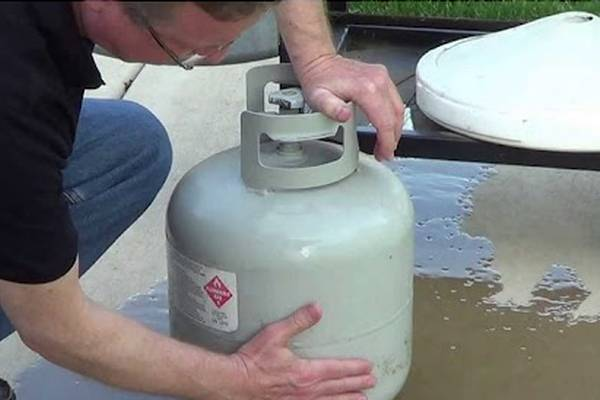# Propane Weigh Guide: How Much Does a Gallon of Propane Weigh?

When you are in an RV, you use propane almost every day. RV users like the benefits but don't know how much propane weighs. When you are figuring out your weight issues, you need to know this figure to help you pack better.

How much does a gallon of propane weigh? A gallon of propane weighs 4.2 pounds. A 20-pound propane tank contains 4.7 gallons of propane. To get the weight of propane in a 20-pound tank, just multiply 4.2 by 4.7 and you will see that a 20-pound propane tank does not hold 20 pounds of propane. It holds 19.74 pounds.

To learn more about the weight of propane in propane tanks, just continue to read our article. It gives you that information and more. Everything weighs something and to meet your weight capacities you need to know the weight of the smallest items.

# What Does Propane Weigh Per Gallon?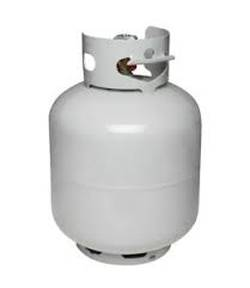The figure we just gave you will answer this question. You are looking at 4.2 pounds per gallon of propane. This number is essential to know as you will not be carrying a full tank of propane at any time.

What we mean by that is that when you do the math, you will find out that 4.2 is beneath the required 5 pounds to make a full 20-pound tank of propane. Of course, you won’t have a full tank because you need to leave room for the gas to expand.

## Does Propane Weigh More Than Water?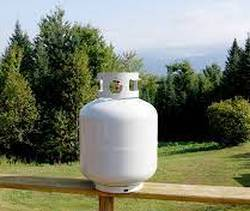No, it doesn’t. In fact, propane weighs about 1/2 as much as water does. A gallon of water weighs approx 8.3 pounds while a gallon of propane weighs in at 4.2 pounds. That means that you will have just under 20 pounds of weight in a propane tank not counting the weight of the tank.

If you have 40 gallons of room you will have 322 pounds approx., of water in your freshwater tank. Two 20-pound tanks of propane will still not come close to that weight as two tanks will only way 39 pounds approx.

## How Much Does a Pound Of Propane Weigh?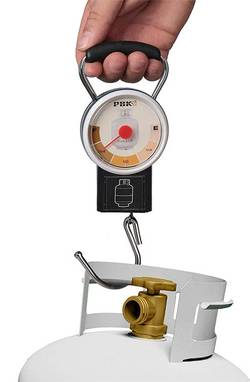A pound of anything is a pound in weight. It is like saying which is heavier a pound of steel or a pound of feathers. A pound is a pound no matter what item it comes in.

The question you want to answer is how many pounds does a 30 or 40-pound propane tank weighs not including the weight of the tank. The answer would depend on how many gallons are inside a 30 or 40-pound tank.

Then you multiply 4.2 by the number of gallons and you get the exact weight when the tank is full.

## How Much Do 7 Gallons Of Propane Weigh?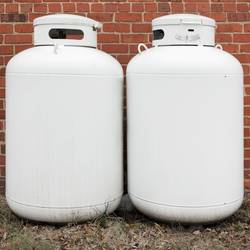This is going to be simple math. 4.2 x 7 equals 29.4 pounds. This number tells you approx. how many gallons you have in a 30-pound propane tank. It is not going to be 30 pounds exactly unless you include the weight of the propane tank. And then it may not be exact.

Each propane tank has what is called the tare weight. That tare weight will be the weight of the pro﻿﻿pan﻿﻿e tank without the propane in it. For most 20-pound tanks that tare weight equals 17 pounds.

19.74 plus 17 equals 36.74 pounds. That should be what your full tank of propane should weigh.

## How Many Gallons Are In a 20 lb Propane Tank?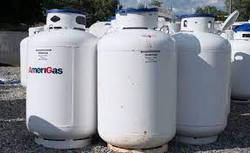They say that 4.7 gallons are what a 20-pound propane tank will have when full. It is not quite 5 gallons as the gas needs room to expand. You may expect more gallons of propane in larger tanks but it is not generally that much more.

A 40-gallon propane tank may only hold 9.4 gallons of propane which is only double of the 20-pound capacity. A 30-pound tank is only holding roughly almost 7 gallons of gas.

However, the 40-pound tank when full will weigh over 70 pounds in total. The other factor you need to figure in your calculations will be cost. Between May and August are the best months to buy propane as that is when it is at its cheapest levels.

## How Much Does a Full 20-gallon Propane Tank Weigh?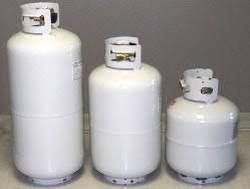When you take the weight of the number of gallons inside the tank and the tare weight of the tank, you should be lifting roughly 36 to 37 pounds of weight. That is 19.74 plus 17 equals 36.74 pounds when the 20-pound propane tank is filled.

Two 20-pound tanks will have you lifting up to almost 74 pounds of weight which is an important number to remember. With every weight you add onto or into your trailer, you inch closer to your tow weight capacity.

That is a mark you do not want to cross.

## How Much Does a 100lb Propane Tank Weigh Full?

A 100-pound propane tank holds roughly 23 gallons of gas. When you multiply that total by 4.2 you get roughly 97 pounds plus the tare weight of the tank. A full 100-pound tank is supposed to weigh 170 pounds.

The cost of filling this size of tank will depend on where you do your propane buying. The cost of propane, like gas, fluctuates from day to day and month to month.

### Some Final Words

When it comes to using an RV or pulling a trailer, weight is everything. To meet all capacities you need to calculate the weight of everything including your propane tanks.

Going over your tow weight may not seem like a big deal but it can be. Something bad may not happen every time but when it does, you are looking at costly repairs. Always check your weights so you and your family are safe.